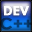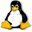Home Latest News Archive Tutorials C++ 2 3 4 Win32 Java OpenGL GLUT OpenGL ES 2 3 4 DirectX VB.NET HTML Photoshop 3dsmax Forums Projects Modelling Links About Us Search Recent Tutorials C / C++ Win32 OpenGL OpenGL ES GLUT## Random Numbers

Tutorials > C++ > Random Numbers

### Introduction

In many applications, you will find it necessary to take some sort of random action eg. in developing a game, you may want an enemy to randomly decide whether he wants to take the path to the left or the path to the right. This tutorial will show you how to generate random numbers.

Contents of main.cpp :

We include the time.h header file as you will see later that we are going to want to request the current time of the computer.

```#include <iostream>
#include <stdlib.h>
#include <time.h>

using namespace std;

int main()
{
```

The function used to generate a random number is the rand function. It takes no parameters and returns an integer between 0 and the constant RAND_MAX. We can assign this value to a variable and print it out. Different computers may generate a different random number.

```	int rnum = rand();
cout << rnum << endl;
```

As mentioned before, a number from 0 to RAND_MAX can be returned. This is displayed below.

```	cout << "Random Number Max Value : ";
cout << RAND_MAX << endl;
```

If you run the above piece of code again, you will notice that every time you run the program, you receive the same random number generated. This may not be what you are looking for as the user will receive the same outcome every time the program is run, therefore defeating the purpose of the randomness.

To fix this, we can use the srand function. It takes one integer parameter. This function seeds the random number generator which will allow it to generate a different set of random numbers.

If you always seed the generator with a value of eg. 4, you will always receive the same set of numbers. If you however use a value of eg. 5, you will receive a different set of numbers.

The idea is therefore to pass a different value to the srand function every time the program is run. We cannot pass a random number to it as the random number will always be the same as was shown above. This is where the system's current time can come in handy.

A time function is available which allows you to return the system's current time. It takes one parameter which is a time_t structure for holding information about the system's current time. If you pass NULL onto the function, the value is only returned. This is all we need and we therefore pass NULL onto the function. We cast the result as unsigned to avoid compiler warnings. You may find that if you run the program a number of times, that the values are close to each other. If you pass time(NULL) * time(NULL) to the rand function, you may get more satisfactory results.

```	srand((unsigned)time(NULL));

rnum = rand();
cout << rnum << endl;
```

You may not want a value from 0 to RAND_MAX. You can generate a number between 0 and some integer value by simply using :

value = rand() % (upper_limit + 1);

```	// Number from 0 to 25
cout << rand() % 26 << endl;
```

If you want the lower limit of the range to be above 0, you need to take the range length + 1 as the mod value and then add the lower limit at the end eg.

value = rand() % (upper_limit - lower_limit + 1) + lower_limit;

```	// Number from 10 to 20
cout << rand() % (20 - 10 + 1)
+ 10 << endl;
```

You may find a situation where you want to find a random number between 0 and 1. To do this you can simply cast the value returned by rand to a float and divide the result by RAND_MAX.

```	// Float between 0 and 1
cout << (float)rand() / RAND_MAX << endl;

system("pause");

return 0;
}```

Congratulations. You should now be able to generate random numbers and you should know how to seed the random number generator to allow an unknown set of numbers to be generated each time your program is run. You should also be able to generate a number that falls within a certain range.

Please let me know of any comments you may have : Contact Me

 Source Files :